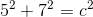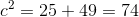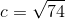# Intermediate Geometry : How to find the length of the hypotenuse of an acute / obtuse triangle

## Example Questions

### Example Question #1 : How To Find The Length Of The Hypotenuse Of An Acute / Obtuse Triangle

An acute scalene triangle has one side length ofinches and another ofinches. Find the length of the hypotenuse.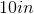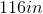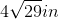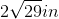Explanation:

To find the length of the hypotenuse, apply the Pythagorean Theorem:, where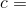the length of the hypotenuse.

Thus, the solution is: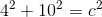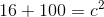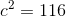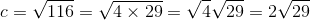### Example Question #2 : How To Find The Length Of The Hypotenuse Of An Acute / Obtuse Triangle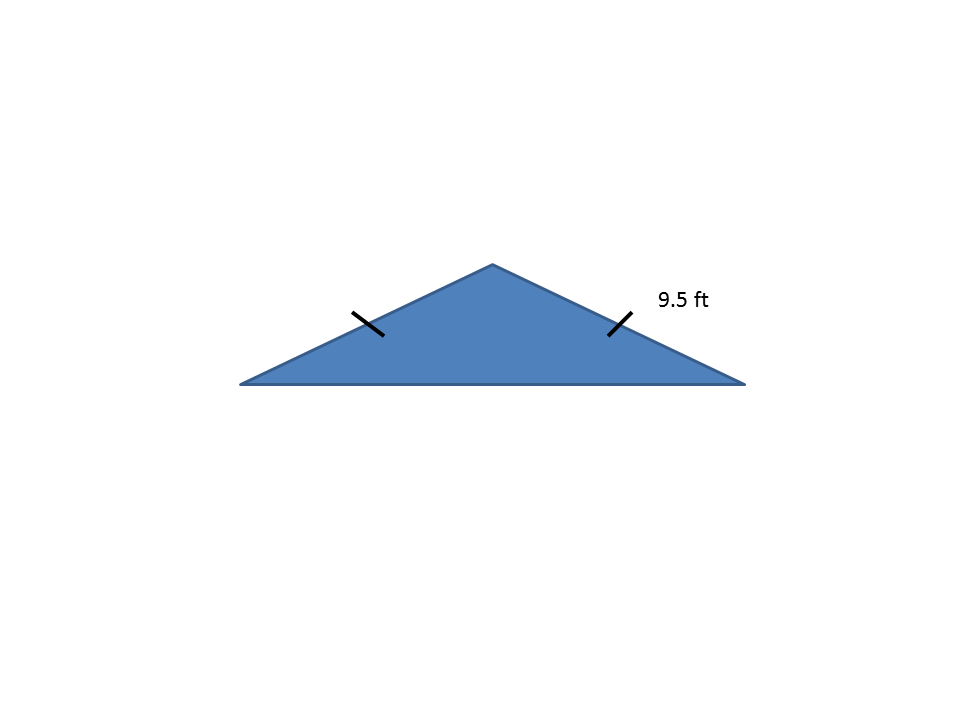Find the hypotenuse of the obtuse isosceles triangle shown above.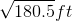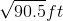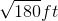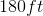Explanation:

To find the length of the hypotenuse, apply the Pythagorean Theorem:, wherethe length of the hypotenuse.

Thus, the solution is: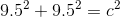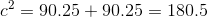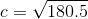### Example Question #3 : How To Find The Length Of The Hypotenuse Of An Acute / Obtuse Triangle

A scalene triangle has one side length ofyards and another side length ofyards. Find the hypotenuse.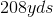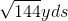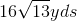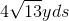Explanation:

To find the length of the hypotenuse, apply the Pythagorean Theorem:, wherethe length of the hypotenuse.

Thus, the solution is: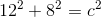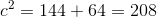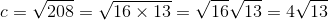### Example Question #4 : How To Find The Length Of The Hypotenuse Of An Acute / Obtuse Triangle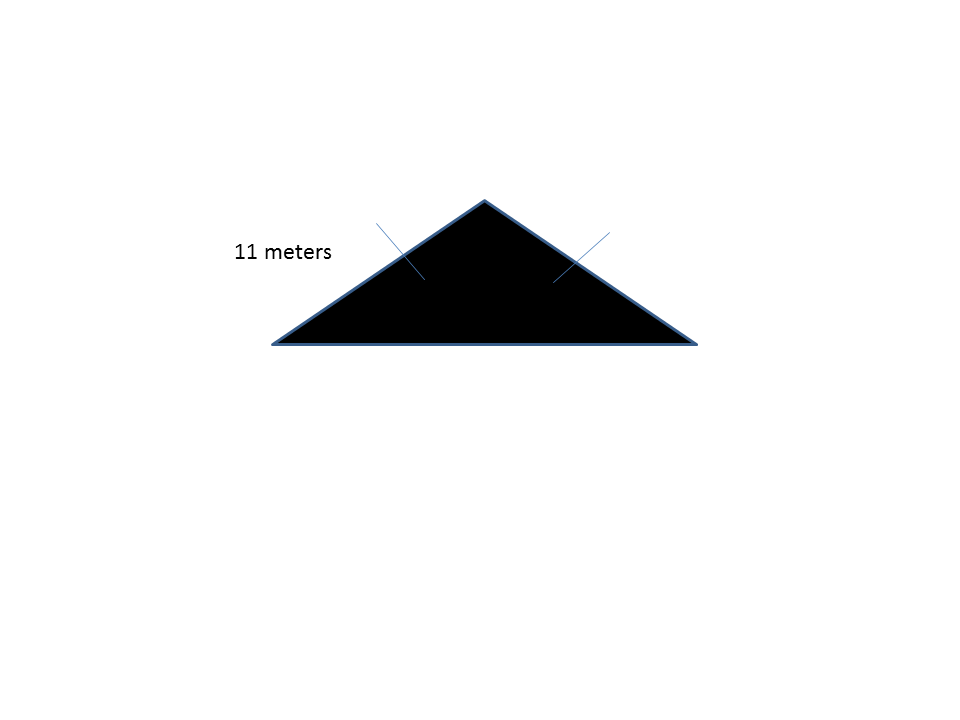Find the hypotenuse of the obtuse isosceles triangle shown above.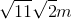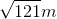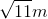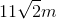Explanation:

To find the length of the hypotenuse, apply the Pythagorean Theorem:, wherethe length of the hypotenuse.

Thus, the solution is: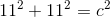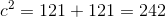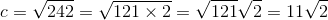### Example Question #5 : How To Find The Length Of The Hypotenuse Of An Acute / Obtuse Triangle

An isosceles right triangle has two sides with a length ofinches each. Find the length of the hypotenuse.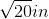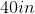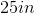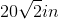Explanation:

To find the length of the hypotenuse, apply the Pythagorean Theorem:, wherethe length of the hypotenuse.

Thus, the solution is: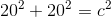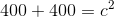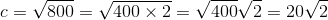### Example Question #6 : How To Find The Length Of The Hypotenuse Of An Acute / Obtuse Triangle

A scalene right triangle has one side length ofinches and another side length ofinches. Find the length of the hypotenuse.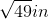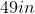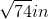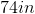Explanation:

To find the length of the hypotenuse, apply the Pythagorean Theorem:, wherethe length of the hypotenuse.

Thus, the solution is: